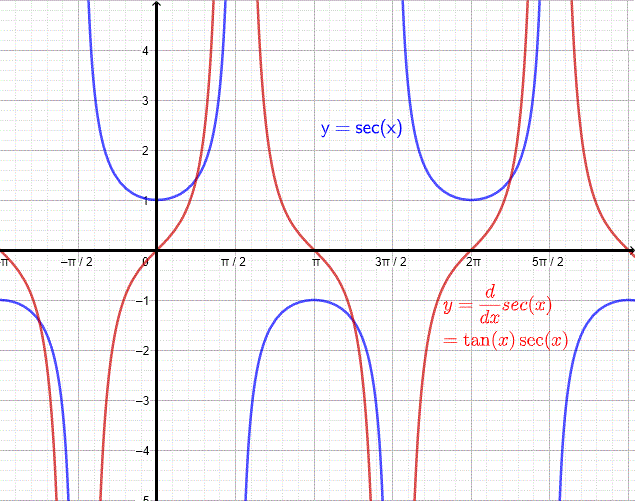# Derivative of sec x

The derivative of $\sec (x)$ is calculated using the quotient rule of derivatives.

## Proof of the Derivative of sec x

A trigonometric identity relating $\sec x$ and $\cos x$ is given by $\sec x = \dfrac { 1 }{ \cos x }$ We use the quotient rule of differentiation to find the derivative of $\sec x$; hence
$\displaystyle { \dfrac {d}{dx} \sec x = \dfrac {d}{dx} (\dfrac{ 1 }{\cos x}) = \dfrac { (\dfrac {d}{dx}1) { \cos x } - 1 (\dfrac {d}{dx} \cos x) } {\cos^2 x} }$

The derivative of the constant 1 is equal to zero. Use the formulae for the derivative of the trigonometric functions $\cos x$ given by $\dfrac {d}{dx}\cos x = - \sin x$ and substitute to obtain

$\displaystyle {\dfrac {d}{dx} \sec x = \dfrac{ (0 - (-\sin x) )}{\cos^2 x}}$

Simplify

$\displaystyle {= \dfrac{ \sin x } {\cos^2 x} = \dfrac{ \sin x }{\cos x} \dfrac{ 1 }{\cos x} = \tan x \sec x}$

conclusion
$\displaystyle {\dfrac {d}{dx} \sec x = \tan x \; \sec x}$

## Graph of sec x and its Derivative

The graphs of $\sec(x)$ and its derivative are shown below.## Derivative of the Composite Function sec (u(x))

We now consider the composite function sec of another function u(x). Use the chain rule of differentiation to write

$\displaystyle \dfrac{d}{dx} \sec (u(x)) = (\dfrac{d}{du} \sec u) (\dfrac{d}{dx} u )$

Simplify

$= \tan u \sec u \dfrac{d}{dx} u$

Conclusion

$\displaystyle \dfrac{d}{dx} \sec (u(x)) = \tan u \; \sec u \; \dfrac{d}{dx} u$

Example 1
Find the derivative of the composite sec functions

1. $f(x) = \sec (x^2 + x - 1)$
2. $g(x) = \sec (\sin(x))$
3. $h(x) = \sec (\sqrt{x+2})$

Solution to Example 1

1. Let $u(x) = x^2 + x - 1$ and therefore $\dfrac{d}{dx} u = \dfrac{d}{dx} (x^2 + x - 1) = 2x + 1$ and apply the rule for the composite sec function given above

$\displaystyle \dfrac{d}{dx} f(x) =\tan u \sec u \dfrac{d}{dx} u = \tan (x^2 + x - 1) \sec (x^2 + x - 1) \times (2x + 1)$

$= (2x + 1) \; \tan (x^2 + x - 1) \; \sec (x^2 + x - 1)$

2. Let $u(x) = \sin x$ and therefore $\dfrac{d}{dx} u = \dfrac{d}{dx} \sin x = \cos x$ and apply the above rule

$\displaystyle \dfrac{d}{dx} g(x) = \tan u \sec u \dfrac{d}{dx} u = \tan (\sin x) \sec (\sin x) \times (\cos x)$

$= \cos x \; \tan (\sin x) \; \sec (\sin x)$

3. Let $u(x) = \sqrt{x+2}$ and therefore $\dfrac{d}{dx} u = \dfrac{1}{2\sqrt{x+2}}$ and apply the rule obtained above

$\displaystyle \dfrac{d}{dx} h(x) = \tan u \sec u \dfrac{d}{dx} u = \tan (\sqrt{x+2}) \sec (\sqrt{x+2}) \times \dfrac{1}{2\sqrt{x+2}}$

$= \dfrac {\tan (\sqrt{x+2}) \; \sec (\sqrt{x+2})} {{2\sqrt{x+2}} }$